# Test: Graphs- 2

Test Description

## 20 Questions MCQ Test GATE Computer Science Engineering(CSE) 2023 Mock Test Series | Test: Graphs- 2

Test: Graphs- 2 for Computer Science Engineering (CSE) 2023 is part of GATE Computer Science Engineering(CSE) 2023 Mock Test Series preparation. The Test: Graphs- 2 questions and answers have been prepared according to the Computer Science Engineering (CSE) exam syllabus.The Test: Graphs- 2 MCQs are made for Computer Science Engineering (CSE) 2023 Exam. Find important definitions, questions, notes, meanings, examples, exercises, MCQs and online tests for Test: Graphs- 2 below.
Solutions of Test: Graphs- 2 questions in English are available as part of our GATE Computer Science Engineering(CSE) 2023 Mock Test Series for Computer Science Engineering (CSE) & Test: Graphs- 2 solutions in Hindi for GATE Computer Science Engineering(CSE) 2023 Mock Test Series course. Download more important topics, notes, lectures and mock test series for Computer Science Engineering (CSE) Exam by signing up for free. Attempt Test: Graphs- 2 | 20 questions in 60 minutes | Mock test for Computer Science Engineering (CSE) preparation | Free important questions MCQ to study GATE Computer Science Engineering(CSE) 2023 Mock Test Series for Computer Science Engineering (CSE) Exam | Download free PDF with solutions
 1 Crore+ students have signed up on EduRev. Have you?
Test: Graphs- 2 - Question 1

### Which of the following algorithms can be used to most efficiently determine the presence of a cycle in a given graph ?

Test: Graphs- 2 - Question 2

### Traversal of a graph is different from tree because

Test: Graphs- 2 - Question 3

### What are the appropriate data structures for following algorithms? 1) Breadth First Search 2) Depth First Search 3) Prim's Minimum Spanning Tree 4) Kruskal' Minimum Spanning Tree

Detailed Solution for Test: Graphs- 2 - Question 3

1) Breadth First Search uses Queue
2) Depth First Search uses Stack
3) Prim's Minimum Spanning Tree uses Priority Queue.
4) Kruskal' Minimum Spanning Tree uses Union Find.

Test: Graphs- 2 - Question 4

The Breadth First Search algorithm has been implemented using the queue data structure. One possible order of visiting the nodes of the following graph is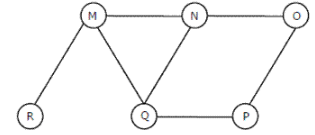Detailed Solution for Test: Graphs- 2 - Question 4

Option (A) is MNOPQR. It cannot be a BFS as the traversal starts with M, but O is visited before N and Q. In BFS all adjacent must be visited before adjacent of adjacent. Option (B) is NQMPOR. It also cannot be BFS, because here, P is visited before O. (C) and (D) match up to QMNP. We see that M was added to the queue before N and P (because M comes before NP in QMNP). Because R is M's neighbor, it gets added to the queue before the neighbor of N and P (which is O). Thus, R is visited before O.

Test: Graphs- 2 - Question 5

Let G be an undirected graph. Consider a depth-first traversal of G, and let T be the resulting depth-first search tree. Let u be a vertex in G and let v be the first new (unvisited) vertex visited after visiting u in the traversal. Which of the following statements is always true? (GATE CS 2000)

Detailed Solution for Test: Graphs- 2 - Question 5

In DFS, if 'v' is visited after 'u', then one of the following is true.

1) (u, v) is an edge.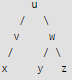2) 'u' is a leaf node.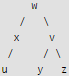In DFS, after visiting a node, we first recur for all unvisited children. If there are no unvisited children (u is leaf), then control goes back to parent and parent then visits next unvisited children.

Test: Graphs- 2 - Question 6

Consider the following graph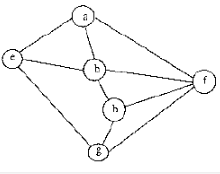Among the following sequences
I) a b e g h f
II) a b f e h g
III) a b f h g e
IV) a f g h b e

Q. Which are depth first traversals of the above graph?

Detailed Solution for Test: Graphs- 2 - Question 6

We can check all DFSs for following properties.

In DFS, if a vertex 'v' is visited after 'u', then one of the following is true.

1) (u, v) is an edge.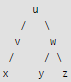2) 'u' is a leaf node.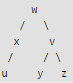In DFS, after visiting a node, we first recur for all unvisited children. If there are no unvisited children (u is leaf), then control goes back to parent and parent then visits next unvisited children.

Test: Graphs- 2 - Question 7

Make is a utility that automatically builds executable programs and libraries from source code by reading files called makefiles which specify how to derive the target program. Which of the following standard graph algorithms is used by Make.

Detailed Solution for Test: Graphs- 2 - Question 7

Make can decide the order of building a software using topological sorting. Topological sorting produces the order considering all dependencies provide by makefile. See following for details. Topological Sorting

Test: Graphs- 2 - Question 8

Given two vertices in a graph s and t, which of the two traversals (BFS and DFS) can be used to find if there is path from s to t?

Detailed Solution for Test: Graphs- 2 - Question 8

We can use both traversals to find if there is a path from s to t.

Test: Graphs- 2 - Question 9

Which of the following condition is sufficient to detect cycle in a directed graph?

Test: Graphs- 2 - Question 10

Is following statement true/false If a DFS of a directed graph contains a back edge, any other DFS of the same graph will also contain at least one back edge

Detailed Solution for Test: Graphs- 2 - Question 10

A back edge means a cycle in graph. So if there is a cycle, all DFS traversals would contain at least one back edge.

Test: Graphs- 2 - Question 11

Is following statement true/false? A DFS of a directed graph always produces the same number of tree edges, i.e., independent of the order in which vertices are considered for DFS.

Detailed Solution for Test: Graphs- 2 - Question 11

Consider the following graph. If we start from 'a', then there is one tree edge. If we start from 'b', then there is no tree edge. a---->b

Test: Graphs- 2 - Question 12

If the DFS finishing time f[u] > f[v] for two vertices u and v in a directed graph G, and u and v are in the same DFS tree in the DFS forest, then u is an ancestor of v in the depth first tree.

Detailed Solution for Test: Graphs- 2 - Question 12

In a graph with three nodes, r u and v, with edges (r; u) and (r; v), and r is the starting point for the DFS, u and v are siblings in the DFS tree, neither as the ancestor of the other.

Test: Graphs- 2 - Question 13

Consider the DAG with Consider V = {1, 2, 3, 4, 5, 6}, shown below. Which of the following is NOT a topological ordering?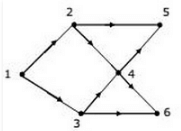Detailed Solution for Test: Graphs- 2 - Question 13

In option D, 1 appears after 2 and 3 which is not possible in Topological Sorting. In the given DAG it is directly visible that there is an outgoing edge from vertex 1 to vertex 2 and 3 hence 2 and 3 cannot come before vertex 1 so clearly option D is incorrect topological sort. But for questions in which it is not directly visible we should know how to find topological sort of a DAG.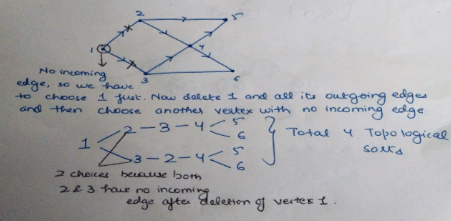Test: Graphs- 2 - Question 14

Let G be a graph with n vertices and m edges. What is the tightest upper bound on the running time on Depth First Search of G? Assume that the graph is represented using adjacency matrix.

Detailed Solution for Test: Graphs- 2 - Question 14

Depth First Search of a graph takes O(m+n) time when the graph is represented using adjacency list. In adjacency matrix representation, graph is represented as an "n x n" matrix. To do DFS, for every vertex, we traverse the row corresponding to that vertex to find all adjacent vertices (In adjacency list representation we traverse only the adjacent vertices of the vertex). Therefore time complexity becomes O(n2)

Test: Graphs- 2 - Question 15

Consider the tree arcs of a BFS traversal from a source node W in an unweighted, connected, undirected graph. The tree T formed by the tree arcs is a data structure for computing.

Detailed Solution for Test: Graphs- 2 - Question 15

BFS always produces shortest path from source to all other vertices in an unweighted graph.

Test: Graphs- 2 - Question 16

Suppose depth first search is executed on the graph below starting at some unknown vertex. Assume that a recursive call to visit a vertex is made only after first checking that the vertex has not been visited earlier. Then the maximum possible recursion depth (including the initial call) is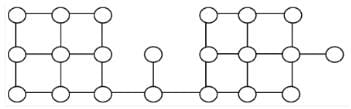Detailed Solution for Test: Graphs- 2 - Question 16

The following diagram shows the worst case situation where the recursion tree has maximum depth.So the recursion depth is 19 (including the first node).

Test: Graphs- 2 - Question 17

Let T be a depth first search tree in an undirected graph G. Vertices u and n are leaves of this tree T. The degrees of both u and n in G are at least 2. which one of the following statements is true?

Detailed Solution for Test: Graphs- 2 - Question 17

Below example shows that A and B are FALSE: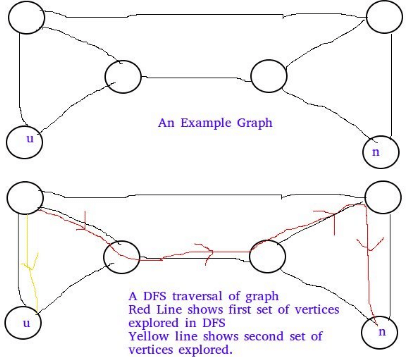Below example shows C is false: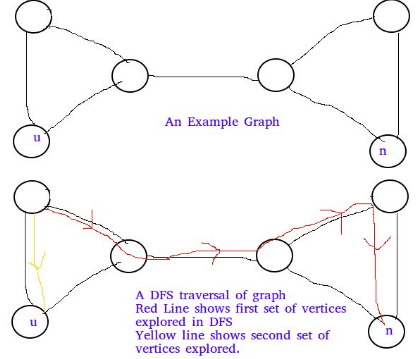Test: Graphs- 2 - Question 18

Let G be an undirected graph. Consider a depth-first traversal of G, and let T be the resulting depth-first search tree. Let u be a vertex in G and let v be the first new (unvisited) vertex visited after visiting u in the traversal. Which of the following statements is always true?

Detailed Solution for Test: Graphs- 2 - Question 18

In DFS, if 'v' is visited after 'u', then one of the following is true.
1) (u, v) is an edge.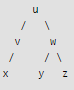2) 'u' is a leaf node.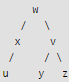In DFS, after visiting a node, we first recur for all unvisited children. If there are no unvisited children (u is leaf), then control goes back to parent and parent then visits next unvisited children.

Test: Graphs- 2 - Question 19

In a depth-first traversal of a graph G with n vertices, k edges are marked as tree edges. The number of connected components in G is

Detailed Solution for Test: Graphs- 2 - Question 19

Tree edges are the edges that are part of DFS tree.  If there are x tree edges in a tree, then  x+1 vertices in the tree. The output of DFS is a forest if the graph is disconnected.  Let us see below simple example where graph is disconnected.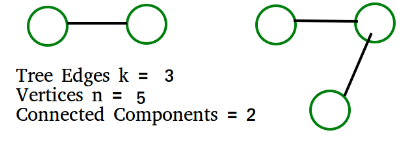The above example matches with D option More Examples: 1) All vertices  of Graph are connected.  k must be n-1.  We get number of connected components  = n- k =  n - (n-1) = 1 2) No vertex is connected. k must be 0.  We get number of connected components  = n- k =  n - 0 = n

Test: Graphs- 2 - Question 20

Consider the following directed graph.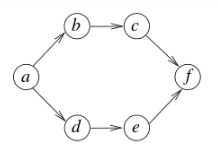Q. The number of different topological orderings of the vertices of the graph is

Detailed Solution for Test: Graphs- 2 - Question 20

Following are different 6 Topological Sortings

a-b-c-d-e-f
a-d-e-b-c-f
a-b-d-c-e-f
a-d-b-c-e-f
a-b-d-e-c-f
a-d-b-e-c-f

## GATE Computer Science Engineering(CSE) 2023 Mock Test Series

149 docs|215 tests
 Use Code STAYHOME200 and get INR 200 additional OFF Use Coupon Code
Information about Test: Graphs- 2 Page
In this test you can find the Exam questions for Test: Graphs- 2 solved & explained in the simplest way possible. Besides giving Questions and answers for Test: Graphs- 2, EduRev gives you an ample number of Online tests for practice

## GATE Computer Science Engineering(CSE) 2023 Mock Test Series

149 docs|215 tests